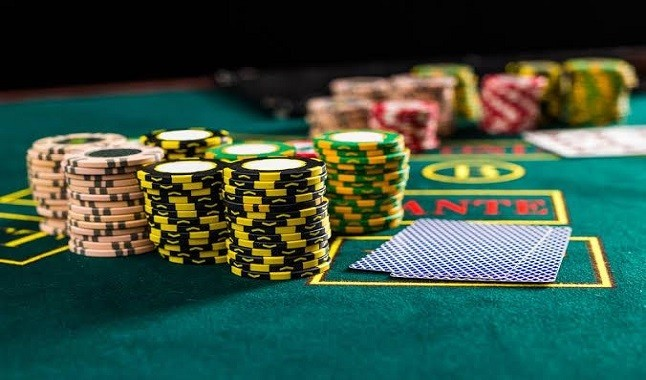This site uses cookies. When you browse the site you are consenting to its use. Know more.

# Learning how to calculate pot oddsIn this article, we will learn, in a simple and practical way, how to calculate pot odds.

Poker, as we well know, is a game that mixes logic, psychology, probability and mathematics. Therefore, it is important that we never neglect the mathematical aspects of the sport, which is why it is essential that we know how to correctly calculate the pot odds.

It seems very visible, that many players neglect the mathematical part for supposing that it is complex or difficult to understand. Perhaps, in high-level games, in fact, there are complexities. But the truth is that in the vast majority of games that you will participate in, the basics serve to give you a great advantage against the field.

It should be noted that pot odds are the ratio, or the relationship, between the size of the pot (total value), and the bet you must pay.

Understanding and knowing how to properly calculate pot odds is vital for any poker player, regardless of the levels at which he plays. It is a basic concept of the game, which will help you find out whether your expectation of making money in the long run is positive or negative. It will certainly help to simplify many of the spots that will appear in the course of your poker career.

After explaining what pot odds are, we give you the formula that should be used:

(VALUE OF YOUR CALL) / (VALUE OF THE POT = THE BET OF YOUR OPPONENT + THE VALUE OF YOUR CALL) = POT ODDS.

With a practical example, it will certainly be easier:

Let's assume that the pot has 1500 chips and the opponent makes a bet of 700. To calculate the pot odds, just divide the bet size by the pot size, that is, the sum of what is already on the table, the opponent's bet and the value of your call.

In this case, the calculation would be 700/2900, which would give approximately 0.24 or 24%.

As mentioned at the beginning of the article, simple, practical and vital to the success of any player, regardless of the games and levels they are playing.

Daniel Dornelles

7 Tips to evolve in Poker

Share "Learning how to calculate pot odds" via: